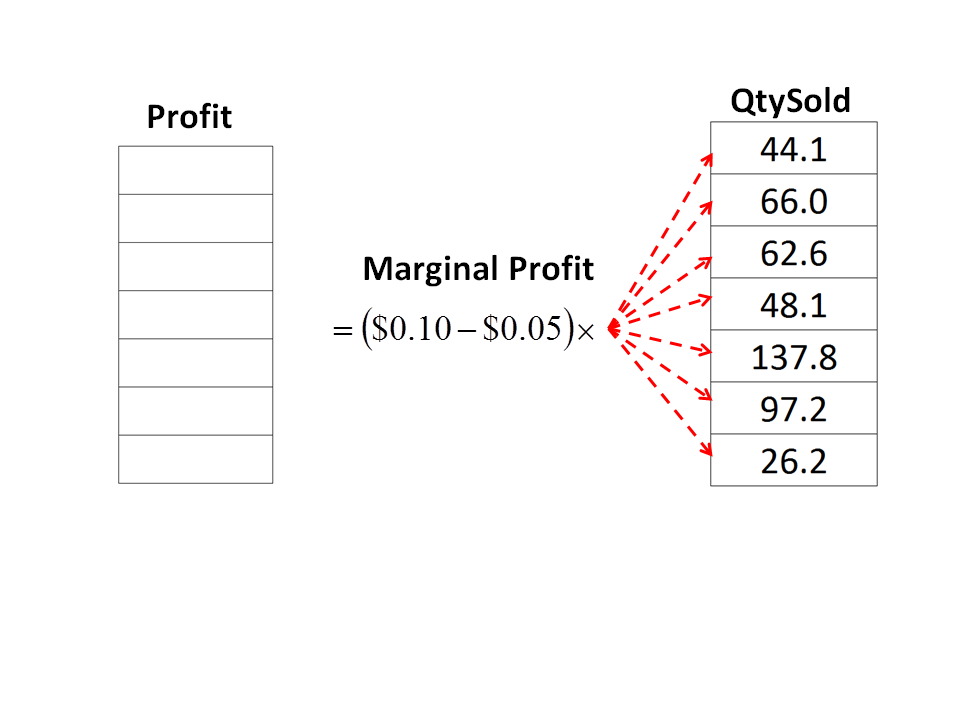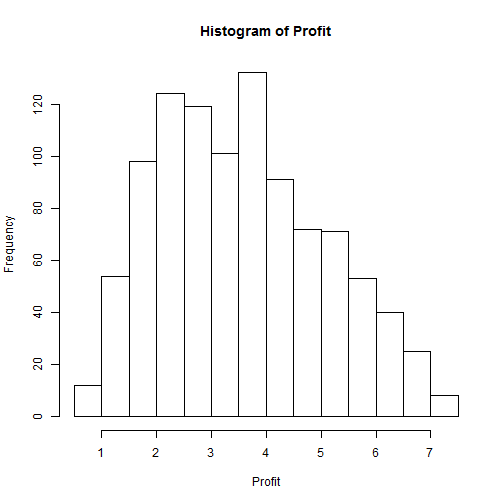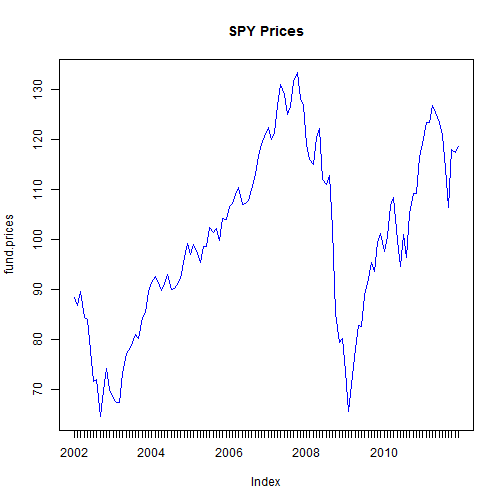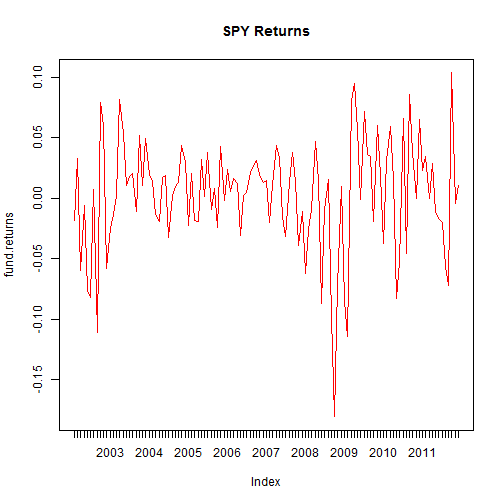# Monte Carlo Simulation in R: Introduction

SE350 Team

### Two Methods:

• Instantly produce all of your Random Variables and store them in a data structure (usually a vector for simple models or a matrix for complex models)
• Iteratively produce random variables and conduct necessary model calculations from inside of a for loop

$Profit=(Price-Cost)*QtySold$

Where

• price=$0.10 • cost=$0.05
• The quantity sold per week is a triangular distribution with a low of 10, a high of 150, and a most likely value of 50### Creating a Vector From Triangular Distribution

We can create a vector of thousands or millions of triangular random variables using the triangle package. After installing this package, use it as seen below (a=minimum, b=maximum, c=mode):

library(triangle)
QtySold<-rtriangle(n=1000,a=10,b=150,c=50)


We have just created 1000 random triangular variables. If I wanted to look at the first 5 variables:

QtySold[1:5]  ##First 5 RV's

  69.47  72.00  73.94 124.61  64.42


### Understanding Use of Vectors for MC:### Lemon Aid Problem with Vectors

Now watch how simple it is to conduct your Monte Carlo Calculations in R:

Profit<-(0.10-0.05)*QtySold


Does it get any easier than this!!!!!!

### Analyzing Output: Histogram

hist(Profit)### Analyzing Output: Summary Data

summary(Profit)

   Min. 1st Qu.  Median    Mean 3rd Qu.    Max.
0.601   2.400   3.470   3.550   4.700   7.380


### Calculating Probability

##Find how many iterations are over $5 length(Profit[Profit>5])/1000   0.197  What this code is doing is creating a vector of only those values that are greater than$5, and then measuring the length of this new vector.

### Monte Carlo Simulation with Loop:

If we loop through each of our iterations, produce a random variable, conduct any necessary calculations, and store the answer, then we have accomplished the same thing we discussed above. As problems get more comlicated, you are often forced to use a loop to run a monte carlo simulation.

You could therefore solve the lemon aid monte carlo simulation problem with the following code:

Profit=NULL   ##instantiate object
for(i in 1:1000) {  ##loop 1000 times
QtySold<-rtriangle(n=1,a=10,b=150,c=50)
Profit[i]<-(0.10-0.05)*QtySold
}


### Bonus: Financial Analysis in R:

library(zoo)
library(tseries)
fund.prices<-get.hist.quote(instrument="SPY",
start="2002-01-01",  end="2011-12-31",
origin="1970-01-01",  compression="m",
retclass="zoo",quiet=TRUE)


Change them from daily to monthly

index(fund.prices) = as.yearmon(index(fund.prices))


Calculate returns

fund.returns = diff(log(fund.prices))


### Bonus: Financial Plots (1 of 2):

plot(fund.prices,main="SPY Prices",col="blue")### Bonus: Financial Plots (2 of 2):

plot(fund.returns,main="SPY Returns",col="red")